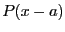Next: Gerschgorin circles Up: Kharitonov polynomials Previous: Kharitonov polynomials   Contents

Implementation

The following procedure allows to determine if a polynomial has a real root within a given range:

int Kharitonov(int Degree,
INTERVAL_VECTOR (* TheCoeff)(double a,INTERVAL_VECTOR &),
int (* Solve_Poly)(double *, int *,double *),
INTERVAL_VECTOR &Input)
where:
• Degree: the degree of the polynomial
• TheCoeff: a procedure that allows to compute the interval coefficients of the polynomial at a point a (i.e. the coefficients of the polynomial)
• Solve_Poly: a procedure that compute the real part of the roots of a polynomial. It takes as argument the coefficients of the polynomial, a pointer to an integer that is initially the degree of the polynomial and the real roots are stored in the last argument. This procedure returns the number of roots or -1 if the computation has failed. ALIAS provides as possible procedure ALIAS_Solve_Poly_PR.
• Input: the range for the root
Note that there exists an ALIAS-Maple procedure that uses this procedure to generate a simplification procedure, see the ALIAS-Maple manual.

Jean-Pierre Merlet 2012-12-20